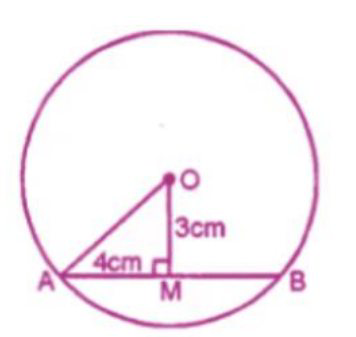# ML Aggarwal Solutions Class 9 Mathematics Solutions for Circle Exercise 15.1 in Chapter 15 - Circle

A chord of length 8 cm is at a distance of 3 cm from the centre of the circle.

Calculate the radius of the circle.

AB is the chord of a circle with center O

And radius OA and OM ⊥ ABAB = 8 cm

OM = 3 cm

OM ⊥ AB

M is the mid-point of AB

AM = ½ AB = ½ × 8 = 4 cm.

Now in right ∆OAM

\begin{aligned} &\mathrm{OA}^{2}=\mathrm{OM}^{2}+\mathrm{AM}^{2}\\ &\text { (By Pythagoras Axiom) }\\ &=(3)^{2}+(4)^{2}=9+16=25\\ &=(5)^{2}\\ &\mathrm{OA}=5 \mathrm{cm} \end{aligned}

Related Questions

Lido

Courses

Teachers

Book a Demo with us

Syllabus

Maths
CBSE
Maths
ICSE
Science
CBSE

Science
ICSE
English
CBSE
English
ICSE
Coding

Terms & Policies

Selina Question Bank

Maths
Physics
Biology

Allied Question Bank

Chemistry
Connect with us on social media!